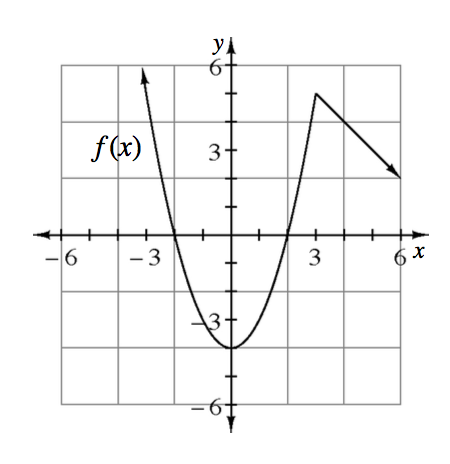### Home > PC3 > Chapter 3 > Lesson 3.1.5 > Problem3-72

3-72.Given the graph of $y=f(x)$, sketch each of the following transformations.

1. $y=-f(x)$

The negative gives all of the $f(x)$-values the opposite sign.
What does this do to the graph?

2. $y=f(x+2)-1$

The $2$ and $-1$ shift the graph. Which directions?

3. $y=\frac{1}{2}f\left(x\right)$

All of the original function values are halved.